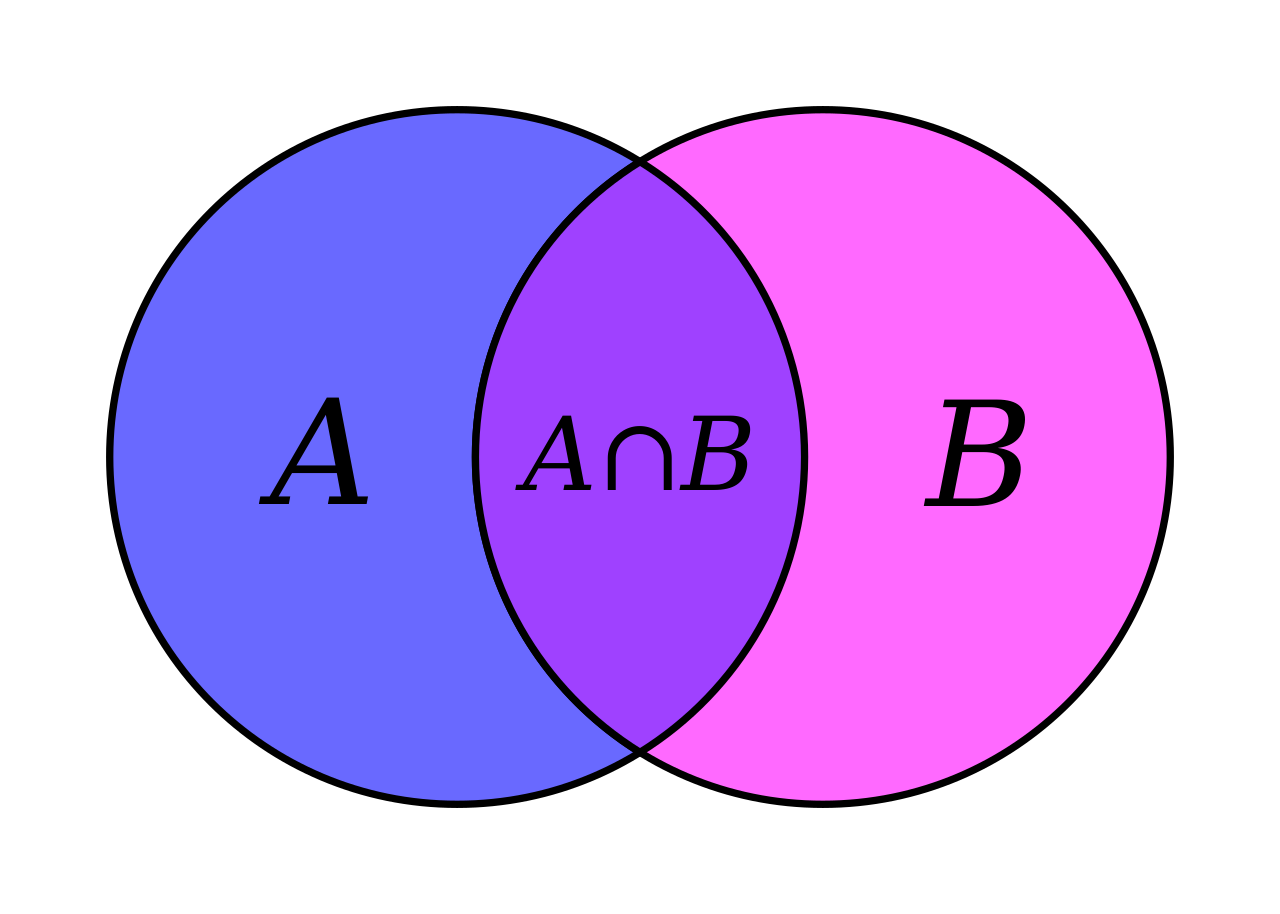# Working with overlapping time periods in PythonFrom https://en.wikipedia.org/wiki/Set_theory

I was working on a project where we had to calculate the total working hours for each employee. If Jessica worked from 11.00 to 12.00 on one task and from 13.00 to 15.00 on another, she had worked three hours. But what if she worked from 10.00 to 15.00 and from 12.00 to 16.00? She could not have worked for nine hours while being in the office for just six hours. However, since people might work on two tasks at the same time, overlaps would not be forbidden.

To solve such a problem — and many others — , we shall implement a specific datastructure which could hold discontinuous time periods and enable to cope with the overlaps.

# The Python standard library

First of all, let’s see what the Python standard library can offer us.

The core of Python’s date and time related functionality is the package. It defines the class, which represents a single moment in time. It also provides us the class, which represents a duration of time (such as four hours). However, as it does not have fixed beginning and end, it does not help us forward with the overlap problem: if you have just and , how would you tell, to which extent they overlap with each other!?

# Hail to strictness

To assure that what we are about the develop meets our actual needs, let’s use the Test Driven Development methodology: we start with defining the requirements as tests and then write the code to get the tests to pass. Writing tests is time consuming and boring, but it helps us to understand what we need and verify that it has indeed been achieved.

So let’s formalize our requirements and start coding! Here is our simple Python module:

`timeperiod/    __init__.py    timeperiod.py    tests.py`

# Instantiation

## The requirements

A can be initialized in three ways:

1. Using objects as its start and end.
2. Using as start and to indicate its duration.
3. An empty shall be initialized with an empty constructor.

Also, cannot be before than .

## The tests

In , let us create our first unittest:

`import unittestfrom datetime import datetimefrom timeset import TimeRangestart = datetime(2021, 5, 20, 12, 12)end = datetime(2021, 5, 20, 14, 12)string_repr = "TimeRange(start=datetime.datetime(2021, 5, 20, 12, 12), end=datetime.datetime(2021, 5, 20, 14, 12))"class TimeRangeInitializationTest(unittest.TestCase):    def test_initialization_with_start_and_end(self):        t = TimeRange(start=start, end=end)        self.assertEqual(str(t), string_repr)    def test_empty_initialization(self):        t = TimeRange()        self.assertEqual(str(t), "TimeRange()")    def test_start_end_integrity(self):        with self.assertRaises(ValueError):            TimeRange(start=end, end=start)if __name__ == '__main__':    unittest.main()`

## The implementation

Now it is time to decide how we are going to store the data internally. Since there might be gaps inside the , simply stroing start and end moments would not be enough. However, each discontinuous period can be broken down into a set of continuous periods.

To reduce the complexity we can make use of the divide et impera approach: if the problem seems too complex to solve, let’s break it down into a set of smaller steps which can be easily accomplished and then combined together to get the end result. In our case the smaller step would be dealing with one continuous time period.

In :

`from dataclasses import dataclassfrom datetime import datetimefrom typing import overload, Optional, Set@dataclass(frozen=True)class ContinuousTimeRange:    start: datetime    end: datetime    def __post_init__(self):        if self.start > self.end:            raise ValueError("Start cannot be greater than end.")class TimeRange:    @overload  # hint for the type checker     def __init__(self):        pass    @overload  # hint for the type checker    def __init__(self, start: datetime, end: datetime):        pass    def __init__(self, start: Optional[datetime] = None, end: Optional[datetime] = None):        self.periods: Set[ContinuousTimeRange] = set()        if start and end:            self.periods.add(ContinuousTimeRange(start, end))        elif start or end:            raise ValueError("A `TimeRange` must have either none or both `start` and `end` specified.")    def __repr__(self) -> str:        return ' + '.join(            [f"TimeRange(start={repr(p.start)}, end={repr(p.end)})" for p in self.periods]        ) or "TimeRange()"`

# Conversion to timedelta and boolean

## The requirements

Just as we can create our object from standard Python s, we should be able to convert it can to the standard types as well: storing data is pretty useless if it cannot be retrieved. We have already implemented the , let’s implement the method and conversion to next.

1. The boolean value of shall indicate whether it’s total duration is greater than zero.
2. The timedelta representation of shall indicate it’s total duration (all gaps excluded).

## The code

Let’s add this to :

`@propertydef as_timedelta(self) -> timedelta:    return self.end - self.start`

And this to :

`def __bool__(self) -> bool:    return len(self._periods) != 0@propertydef as_timedelta(self) -> timedelta:    return sum([p.as_timedelta for p in self._periods])`

# Implementing __contains__()

It would be nice — and useful later on — to be able to check whether a given moment is inside the . Fortunately it is easy to do:

`# timeset.pyclass ContinuousTimeRange:    # all the previous code not shown     def __contains__(self, moment: datetime) -> bool:        return self.start <= moment <= self.endclass TimeRange:    # all the previous code not shown        def __contains__(self, moment: datetime) -> bool:        return any([moment in p for p in self._periods])`

A Buddhist environmentalist software developer